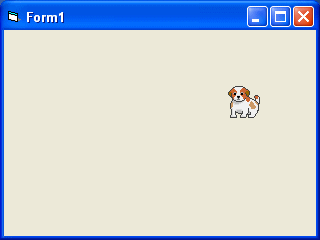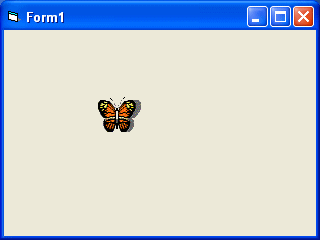# Lesson 32 : Creating Animation using Timer

### 32.1 Animation using Timer

In order animate an object, you need to use a timer. The first step in creating an animation is to drag the timer from the toolbox into the form and set its interval to a certain value other than 0. A value of 1 is 1 milliseconds which means a value of 1000 represents 1 second. The value of the timer interval will determine the speed on an animation.

In the following example, we use a very simple technique to show animation by using the properties Visible=False and Visible=true to show and hide two images alternately. When you click on the program, you should see the following animation.#### The Code

```Private Sub Timer1_Timer()
If Image1.Visible = True Then
Image1.Visible = False
Image2.Visible = True
ElseIf Image2.Visible = True Then
Image2.Visible = False
Image1.Visible = True
End If
End Sub```

Next example shows a complete cycle of a motion such as the butterfly flapping its wing. Previous examples show only manual animation while this example will display an automatic animation once you start the program or by clicking a command button. Similar to the example under lesson 24.2, you need to insert a group of eight images of a butterfly flapping its wings at different stages. Next, insert a timer into the form and set the interval to 10 or any value you like. Remember to make image1 visible while other images invisible at start-up. Finally, insert a command button, rename its caption  as Animate and key in the following statements by double clicking on this button. Bear in mind that you should enter the statements for hiding and showing the images under the timer1_timer subroutine otherwise the animation would work. Clicking on the animate button make timer start ticking and the event will run after every interval of 10 milliseconds or whatever interval you have set at design time. In future lesson, we will show you how to adjust the interval at runtime by using a slider bar or a scroll bar. When you run the program, you should see the following animation:#### The Code

```Private Sub Form_Load()
Image1.Visible = True
x = 0
End Sub

Private Sub Command1_Click()
Timer1.Enabled = True
End Sub

Private Sub Timer1_Timer()
If Image1.Visible = True Then
Image1.Visible = False
Image2.Visible = True

ElseIf Image2.Visible = True Then
Image2.Visible = False
Image3.Visible = True

ElseIf Image3.Visible = True Then
Image3.Visible = False
Image4.Visible = True
ElseIf Image4.Visible = True Then
Image4.Visible = False
Image5.Visible = True
ElseIf Image5.Visible = True Then
Image5.Visible = False
Image6.Visible = True
ElseIf Image6.Visible = True Then
Image6.Visible = False
Image7.Visible = True
ElseIf Image7.Visible = True Then
Image7.Visible = False
Image8.Visible = True
ElseIf Image8.Visible = True Then
Image8.Visible = False
Image1.Visible = True
End If
End Sub```